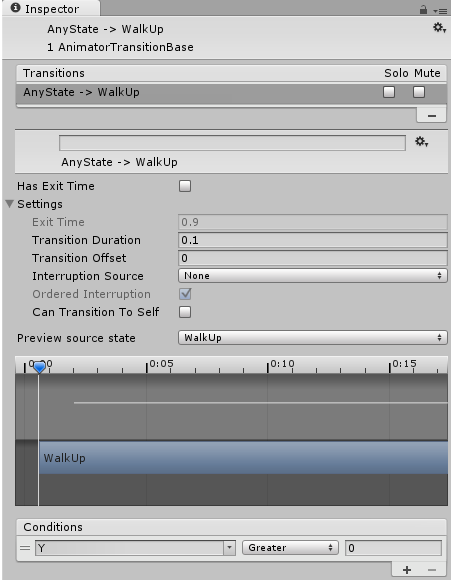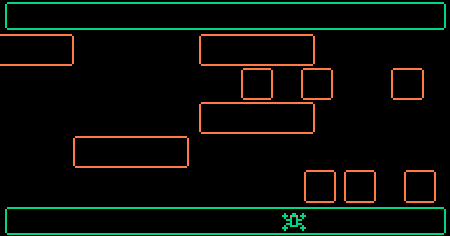# 【Unity3D开发小游戏】青蛙游戏

@[TOC]

## 二、源码

UI资源和源代码请搜索QQ群：1040082875下载

## 三、正文

==Unity5.0.0f4==

### 3.创造青蛙

• 走上去
• 走下
• 向右走
• 向左走 ==注意：每个动画将由两个切片组成。如果我们在一个循环中播放这两个切片，那么我们将有一个动画。==

• 向上(切片0和1)
• 向下(切片2和3)
• 向右(切片4和5)
• 向左(切片6和7)

### 5.青蛙动画状态机

• 向上WalkUp
• 向下WalkDown
• 向右WalkRight
• 向左WalkLeft

``````GetComponent<Animator>().SetFloat("X", 0);
GetComponent<Animator>().SetFloat("Y", 0);
``````

• 如果Y>0然后它在移动向上 (如Y=1，Y=2，Y=3等)
• 如果Y<0然后它在移动向下 (如Y=-1，Y=-2，Y=-3等)
• 如果X>0然后它在移动右边 (如X=1，X=2，X=3等)
• 如果X<0然后它在移动左边 (如X=-1，X=-2，X=-3等)注意：我们还禁用了Can Transition to Self属性防止动画始终重新启动。

• 如果Y<0，从任何状态到向下
• 如果X<0，从任何状态到向左
• 如果X>0， 从任何状态到向右

### 7.青蛙移动脚本

``````using UnityEngine;
using System.Collections;

public class Frog : MonoBehaviour {

// FixedUpdate for Physics Stuff
void FixedUpdate () {

}
}
``````

``````using UnityEngine;
using System.Collections;

public class Frog : MonoBehaviour {
// Jump Speed
public float speed = 0.1f;

// Current jump
Vector2 jump = Vector2.zero;

// FixedUpdate for Physics Stuff
void FixedUpdate () {

}
}
``````

``````// Is the Frog currently jumping?
public bool isJumping() {
return jump != Vector2.zero;
}
``````

``````// FixedUpdate for Physics Stuff
void FixedUpdate () {
if (Input.GetKey(KeyCode.UpArrow))
jump = Vector2.up;
else if (Input.GetKey(KeyCode.RightArrow))
jump = Vector2.right;
else if (Input.GetKey(KeyCode.DownArrow))
jump = -Vector2.up; // -up means down
else if (Input.GetKey(KeyCode.LeftArrow))
jump = -Vector2.right; // -right means left
}
``````

``````void FixedUpdate () {
// Currently jumping?
if (isJumping())
{
// Do Stuff...
}
// Otherwise allow for next jump
else
{
if (Input.GetKey(KeyCode.UpArrow))
jump = Vector2.up;
else if (Input.GetKey(KeyCode.RightArrow))
jump = Vector2.right;
else if (Input.GetKey(KeyCode.DownArrow))
jump = -Vector2.up; // -up means down
else if (Input.GetKey(KeyCode.LeftArrow))
jump = -Vector2.right; // -right means left
}
}
``````

``````// Currently jumping?
if (jump != Vector2.zero)
{
// Remember current position
Vector2 pos = transform.position;

// Jump a bit further
transform.position = Vector2.MoveTowards(pos, pos+jump, speed);

// Subtract stepsize from jumpvector
jump -= (Vector2)transform.position-pos;
}
// Otherwise allow for next jump
else
{
if (Input.GetKey(KeyCode.UpArrow))
jump = Vector2.up * jumpSize;
else if (Input.GetKey(KeyCode.RightArrow))
jump = Vector2.right * jumpSize;
else if (Input.GetKey(KeyCode.DownArrow))
jump = -Vector2.up * jumpSize;    // -up means down
else if (Input.GetKey(KeyCode.LeftArrow))
jump = -Vector2.right * jumpSize; // -right means left
}
``````

``````// Animation Parameters
GetComponent<Animator>().SetFloat("X", jump.x);
GetComponent<Animator>().SetFloat("Y", jump.y);
``````

``````GetComponent<Animator>().speed = Convert.ToSingle(isJumping());
``````

``````// FixedUpdate for Physics Stuff
void FixedUpdate () {
// Currently jumping?
if (isJumping())
{
// Remember current position
Vector2 pos = transform.position;

// Jump a bit further
transform.position = Vector2.MoveTowards(pos, pos+jump, speed);

// Subtract stepsize from jumpvector
jump -= (Vector2)transform.position-pos;
}
// Otherwise allow for next jump
else
{
if (Input.GetKey(KeyCode.UpArrow))
jump = Vector2.up;
else if (Input.GetKey(KeyCode.RightArrow))
jump = Vector2.right;
else if (Input.GetKey(KeyCode.DownArrow))
jump = -Vector2.up; // -up means down
else if (Input.GetKey(KeyCode.LeftArrow))
jump = -Vector2.right; // -right means left
}

// Animation Parameters
GetComponent<Animator>().SetFloat("X", jump.x);
GetComponent<Animator>().SetFloat("Y", jump.y);
GetComponent<Animator>().speed = Convert.ToSingle(isJumping());
}
``````

``````void OnCollisionEnter2D(Collision2D coll) {
// Game Over
Destroy(gameObject);
}
``````

### 9.生成车辆

``````using UnityEngine;
using System.Collections;

public class Spawn : MonoBehaviour {
public GameObject prefab;
public float interval = 1;

// Use this for initialization
void Start () {

}
}
``````

``````// Use this for initialization
void Start () {
InvokeRepeating("SpawnNext", 0, interval);
}

void SpawnNext() {
// Instantiate
GameObject g = (GameObject)Instantiate(prefab,
transform.position,
Quaternion.identity);
}
``````

``````using UnityEngine;
using System.Collections;

public class Spawn : MonoBehaviour {
public GameObject prefab;
public float interval = 1;
public Vector2 velocity = Vector2.right;

// Use this for initialization
void Start () {
InvokeRepeating("SpawnNext", 0, interval);
}

void SpawnNext() {
// Instantiate
GameObject g =(GameObject)Instantiate(prefab,
transform.position,
Quaternion.identity);
// Set Velocity
g.GetComponent<Rigidbody2D>().velocity = velocity;
}
}
``````

### 10.平台

``````using UnityEngine;
using System.Collections;

public class Platform : MonoBehaviour {

void OnTriggerStay2D(Collider2D coll) {

}

void OnTriggerExit2D(Collider2D coll) {

}
}
``````

``````using UnityEngine;
using System.Collections;

public class Platform : MonoBehaviour {

void OnTriggerStay2D(Collider2D coll) {
// Frog? Then make it a Child
if (coll.name == "Frog")
coll.transform.parent = transform;
}

void OnTriggerExit2D(Collider2D coll) {
coll.transform.parent = null;
}
}
``````

### 11.水

• 触水
• 不站在站台上
• 不跳

``````using UnityEngine;
using System.Collections;

public class Water : MonoBehaviour {

void OnTriggerStay2D(Collider2D coll) {
// Frog?
if (coll.name == "Frog")
// Not Jumping?
if (!coll.GetComponent<Frog>().isJumping())
// Not on a platform?
if (coll.transform.parent == null)
// Game Over
Destroy(coll.gameObject);
}
}
``````

*注意：一开始，我们会检测碰撞器是否真的碰撞到青蛙。之后，我们通过使用GetComponent().isJump()来判断是否在跳跃状态。然后，我们就会发现它是否在平台上。比如transform.parent是null。如果所有这些都适用，那么我们就消灭青蛙。*### 12.边界

``````using UnityEngine;
using System.Collections;

public class Border : MonoBehaviour {

void OnTriggerEnter2D(Collider2D coll) {
Destroy(coll.gameObject);
}
}
``````

• 一个输赢的屏幕
• 多个级别
• 一个更复杂的艺术风格
• 一个高分。